CHEMISTRY O LEVEL(FORM THREE) – CHEMICAL KINETICS, EQUILIBRIUM AND ENERGETICS RATE OF REACTION EQUILIBRIUM AND ENERGETICS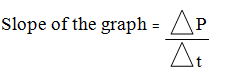Written by

CHEMICAL KINETICS, EQUILIBRIUM AND ENERGETICS

RATE OF REACTION EQUILIBRIUM AND ENERGETICS

1. RATE OF CHEMICAL REACTION
This is the speed at which reaction is proceeding per unit time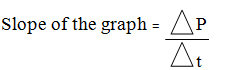It will be positive because product is increasing. The slope gives the rate of reaction
The SI unit = mol dm-3S-1
If the plot is reactant concentration against time the slope will be negative
NB: The units of rate depend on the units of reactant or products used
Example; In an experiment of determining rate, the following volumes of gas in the syringe were recorded in every 10 seconds.
 Time (sec) 0 10 20 30 40 50 60 70 80 Volume (cm3) 0 18 30 40 48 53 57 58 58
a) Plot a graph of the volume shown on the syringe against time
b) Determine the rate of reaction
2. FACTORS AFFECTING RATE OF REACTION
a) Temperature
b) Concentration
c) Catalyst
d) Surface area
e) Pressure (gases)
f) Light

A) TEMPERATURE
The increase in temperature increases the rate of chemical reaction. This is because when temperature increases the particles acquire kinetic energy, hence move faster.
For this matter the collision of the reacting particles also increases.
For every 100c rise the reaction rate doubles
B) CONCENTRATION
The reaction occurs when the reacting substances are allowed to come into contact. The rate of reaction therefore depends on collision frequency which in turn depends on how crowded (concentrated) the particles are
C) SURFACE AREA
The size of particles in heterogeneous reaction influences the rate of chemical reactions. The smaller the particles, the larger the surface area and thus faster rate of reaction
Example; lumps of marble chips (CaCO3) react slowly with dilute hydrochloric acid while the powder of CaCO3 reacts fast with dilute HCl
Aluminium foil slowly reacts with aqueous solution of NaOH when warmed together compared to aluminium powder
D. LIGHT
Light energies some of the reacting molecules (particles). In such reactions, molecules absorb energy in form of light energy rather than heat energy and so they are called photochemical reactions.
Examples are;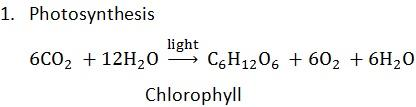2. Decomposition of silver halides on exposure to light
3. Photography
Silver bromide (AgBr) is used in photographic film. It decomposes an exposure to light asWhen light falls on the film, it causes the decomposition of AgBr as shown above. The extent of decomposition depends on the brightness of sun light.
Different amounts of light reflected from the dark and light surfaces darken the AgBr film to different degrees producing a photograph.
E. CATALYST
A catalyst can either slow down or speed up the rate of reaction.
HOW DO CATALYSTS SPEED UP THE RATE OF REACTION?
For the substance to react they must overcome the energy barrier (Activation energy, EA)
If EA is too great, the substances cannot react at room temperature because the particles don’t process enough energy to surmount the barrier. In such cases, the supply energy in form of heat enables the reaction to proceed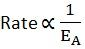1. Exothermic reactions – release heat to the surrounding
2. Endothermic reaction – Absorb heat from the surrounding

Exothermic reaction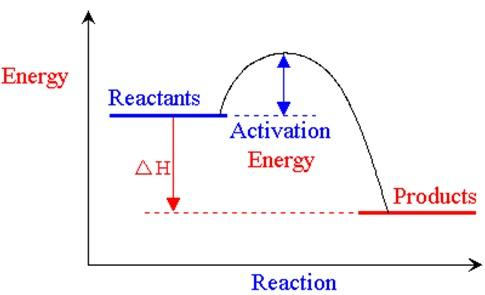Endothermic reaction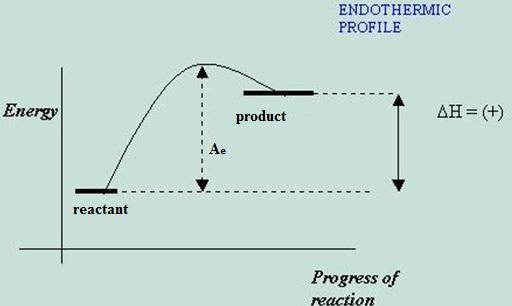EXPLANATIONS.
1. The energy relationship between reactants and products of a chemical reaction.

A catalyst increases reaction rate by lowering activation energy which is a sort of “Energy hump”, thus providing an easier path from reactants to products.
2. PRESSURE
(For reactants in gaseous phase)
The increase in pressure brings the gaseous particles closer and closer together. This in turn increases the collision frequency. The latter increases the rate of reactions.
3. REVERSIBLE AND IRREVERSIBLE REACTIONS
Reversible reactions are reactions which proceed in both directions (background)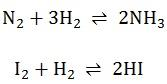IRREVERSIBLE reactions are the reactions which proceed to forward direction only regardless of conditions applied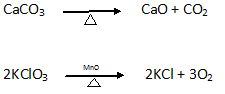4. CHEMICAL EQUILIBRIUM
Chemical equilibrium is the state at which the forward and backward reactions are proceeding at the same rate.
That is there’s some sort of balance between the reactants and products
Chemical equilibrium occurs in a CLOSED SYSTEM.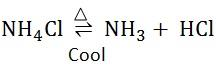Consider the analogous system of compounds in the same phase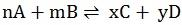As soon as little C and D are formed a reverse reaction will begin. At the beginning the forward reaction will predominate but as C and D accumulate the reverse reaction builds up until an equilibrium is reached (The forward and reserve reaction proceed at the same rate)
The point at which the rate of forward reaction = the rate of backward reaction is called the equilibrium point or position.
Le Chatelier’s principle:
States that: “If a chemical system in equilibrium and one of the factors involved in the equilibrium is changed, the equilibrium will shift so as to minimize the effect of the change”
FACTORS AFFECTING CHEMICAL EQUILIBRIUM
A. TEMPERATURE
The effects of temperature in chemical equilibrium depends on whether chemical reaction is exothermic or endothermic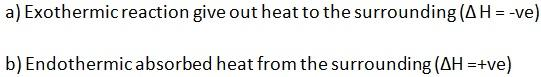In exothermic reactions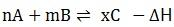When heat is supplied the equilibrium will shift to the direction that requires more heat
I.e. backward reaction
In other words, the effect of heat will be on the forward reaction, thus to minimize the effect the equilibrium must shift backwards.
In endothermic reaction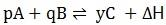The increase in temperature will pose stress on the backward reaction (LHS). So to minimize the effect, the equilibrium must shift to the forward reaction
B. PRESSURE
Pressure affects the equilibrium of reversible reactions involving gases. The increase in pressure causes the equilibrium to shift so as to produce substances occupying less volume or (moles)
Example;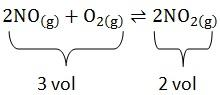Increase in pressure will favour the forward reaction (position of equilibrium will shift to right) as it is accompanied by a decrease in volume. Decrease in pressure will favour backward reaction i.e. position of equilibrium will shift to the left
For the gaseous reaction in which there is no change in volume, pressure has no effect on position of equilibrium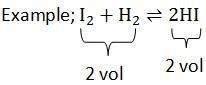Hence if pressure is increased, the position of equilibrium will shift in such a way as to reduce the number of moles present.
The pressure exerted by gases is directly proportional to the number of moles present
C. CONCENTRATION
If concentration of one substance is increased, the reaction will move in a direction to use up the substance whose concentration is increased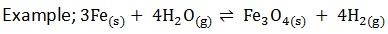What will be the effect of adding more steam to the POE – position of equilibrium of this reaction?
Adding more steam will pose an effect in the equilibrium of the backward reaction. Thus to minimize the effects the reaction must proceed forward therefore the POE will shift to the RHS.
NB: A reaction in which a gas is evolved and allowed to escape or in which a precipitate is formed will proceed in one direction only, even though a reversible reaction might be expected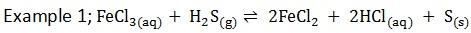S (s) precipitate, it’s almost completely removed from the system. Backward reaction is then not possible
There is complete conversation of reactants to product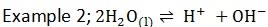H+ are removed as H2 (g) Thus water dissociation has to keep going on
The forward reactions will continue without the backward reactions thus equilibrium is affected
D. CATALYST
A catalyst doesn’t upset the equilibrium of the system because both reactions will proceed at a faster rate
A catalyst doesn’t affect the POE
1. Define the following i) Exothermic reaction
ii) Endothermic reaction
b) State the Le Chatalier’s principle
c) The equation for dissociation of calcium carbonate is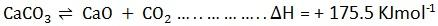What will be the effect on the proportion of calcium carbonate in the equilibrium mixture if
i) Temperature is increased
ii) Pressure is increased
What are the necessary conditions for manufacture of calcium oxide from calcium carbonate in large scale?
i) Increase in temperature the forward reaction is favoured, that is CaCO3 will be splitting to form CaO and O2 thus the effect on the proportion of CaCO3 in the equilibrium will be the decrease of CaCO3.
ii) Increase in pressure
Pressure only affects substances in equilibrium in gaseous phase. Thus, CaCO3 and CaO are considered to have zero volume since they do not react with pressure, but since CO2 reacts with pressure its volume is considered to be 1. Therefore, backward reaction will be favoured thus the effect on the proportion will be the increase in CaCO3
Conditions
• Low pressure
• Large surface area means CaCO3 should be in powdered form
• Concentration
By removing CO2 from the reaction , the concentration of CaO in the products side will favour more quicker forward reaction to replace the lost CO2(g) thus more CaO will be manufactured.
2. Define the following;
i) Reversible reaction
ii) Rate of chemical reaction
iii) Catalyst
b) Bahati attempted to prepare hydrogen gas by reaction between Zn metal with H2SO4 .In this experiment, Zn metal of about 0.5cm diameter was used and 0.2 moles of acid. The rate of formation of hydrogen gas was found to be slow. Explain 3 ways in which the rate of formation of hydrogen could be increased.
c) If bahati wanted 36dm3 of hydrogen at S.T.P. What amount of zinc would be required if 0.2moles of acid were used
The equation for the reaction is;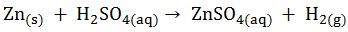i. Decreasing the diameter of zinc so as to increase the surface area.
A large diameter of zinc (o.5cm) yields less amount of hydrogen, thus in order for large amount of hydrogen to be produced, the zinc diameter has to be reduced
ii. Introduce catalyst
iii. Increase concentration of H2SO4
Thus faster reaction and rate.
iv. Increase temperature

Thus increase K.E of the particles so as to taste up the reaction.

5. ENDOTHERMIC AND EXOTHERMIC REACTION.

Exothermic and endothermic reactions are the types of reactions which associate internal energy change to external energy of surrounding environment.

A coldness and hotness in the environmental surrounding after stopping reaction is a result of internal change of energy for reactant to product.

Internal Energy
Internal energy is that energy stored by molecules of chemical substances. When chemical reaction takes place of course influenced by energy changes. The internal energy decreased as a result of giving out, and also the internal energy increases as a result of inlet.

EXOTHERMIC
An exothermic reaction is one during which heat is liberated to the surrounding.

Example; Combustion reaction
The heat change notation represented by change in heat. If quantity heat mentioned in (-ve) sign, Internal energy decreased and that is exothermic reaction.

e.g i. H2(g) + ½O2(g) H2O(l); ΔH = -286KJmol-1

ii. C(s) + O2(g) CO2(g); ΔH = -406KJmol-1

ENDOTHERMIC
An endothermic reaction is one during which heat is absorbed from the surrounding.

Example;
A reaction to form liquid of carbon disulphide which used to manufacture Acetylene gas. Acetylene gas applied in the welding cylinders.

If the quantity heat mentioned by (+ve) sign that is increasing of internal energy. The increasing of internal energy is Endothermic reaction.

e.g i. ½N2(g) + ½O2(g) NO(g); ΔH = +90.3KJmol-1
ii. C(s) + 2S(s) CS2(l); ΔH = +117KJmol-1

ENERGY LEVEL DIAGRAMS

i. EXOTHERMICii. ENDOTHERMICWhere by Ea = Is Activation energy.